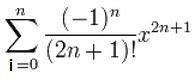# Thread: summation in java using for loops

1.MemberJoin Date
Mar 2011
Posts
27
Rep Power
0

##summation in java using for loops

I am trying to make a summation calculator for the following equation:Where i is the lower limit (in this case it will always be 0) and n is the upper limit (in this problem the user will be asked to enter an integer value of the upper limit).

Here is my code (so far):

import java.io.*;
public class summation
{
public static void main(String args[]) throws IOException
{

int ul;

System.out.print("Enter the upper limit: ");

}

}

I don't understand how to use a for loop to calculate the sum.

After that I got stuck. I appreciate any help i can get thanks! :)
Last edited by java157; 03-16-2011 at 03:48 PM.Reply With Quote

2. ##Originally Posted by java157I am trying to make a summation calculator for the following equation:Are you sure that summation is correct? There is no dependency on i in the term to be summed so the entire thing can be reduced to (n+1)*T where T is that term.

kind regards,

JosReply With Quote

3. ##You will need some math functions.

Math.pow returns the value of the first argument raised to the power of the second argument.
Java Code:
`double Math.pow(double a, double b)`

To calculate the factorial make your own loop e.g.
Java Code:
```private int factorial(int number) {
int counter = number;
while (counter > 1) {
}
}```

And then make a for-loop over your entire equation with your limits put in e.g.
Java Code:
```double totalSum = 0;
double sum = 0;
for (i=0; i<=n; i++) {
...
totalSum += sum;
}

But beware whereever you add an integer to the sum, convert it to a double first, or cast it to a double.
Last edited by ozzyman; 03-16-2011 at 04:57 PM.Reply With Quote

4.MemberJoin Date
Apr 2009
Posts
49
Rep Power
0

##double sum = Math.sin(x); // :-)

that or modify the answer I gave for your other question regarding sums of odd factorials

DavidReply With Quote

####Posting Permissions

• You may not post new threads
• You may not post replies
• You may not post attachments
• You may not edit your posts
•